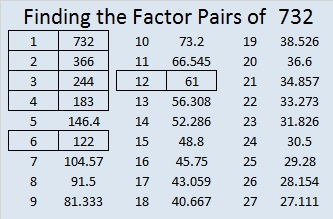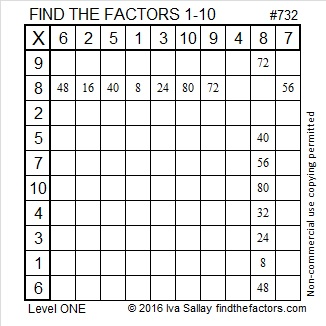# 732 and Level 1

• 732 is a composite number.
• Prime factorization: 732 = 2 x 2 x 3 x 61, which can be written 732 = (2^2) x 3 x 61
• The exponents in the prime factorization are 2, 1, and 1. Adding one to each and multiplying we get (2 + 1)(1 + 1)(1 + 1) = 3 x 2 x 2 = 12. Therefore 732 has exactly 12 factors.
• Factors of 732: 1, 2, 3, 4, 6, 12, 61, 122, 183, 244, 366, 732
• Factor pairs: 732 = 1 x 732, 2 x 366, 3 x 244, 4 x 183, 6 x 122, or 12 x 61
• Taking the factor pair with the largest square number factor, we get √732 = (√4)(√183) = 2√183 ≈ 27.0554985.Finding the factors that solve this factoring puzzle will be easier than that!Print the puzzles or type the solution on this excel file: 10 Factors 2016-01-04

———————————————————

Here are some more facts about the number 732:

732 is the sum of consecutive numbers several different ways:

• 243 + 244 + 245 = 732; that’s 3 consecutive numbers.
• 88 + 89 + 90 + 91 + 92 + 93 + 94 + 95 = 732; that’s 8 consecutive numbers.
• 19 + 20 + 21 + 22 + 23 + 24 + 25 + 26 + 27 + 28 + 29 + 30 + 31 + 32 + 33 + 34 + 35 + 36 + 37 + 38 + 39 + 40 + 41 + 42 = 732; that’s 24 consecutive numbers.

732 is the sum of the 8 prime numbers from 73 to 107. It is also the sum of the 10 prime numbers from 53 to 97. Do you know what all those prime numbers are?

Because 61 is one of its factors, 732 is the hypotenuse of Pythagorean triple 132-720-732. That means 132² + 720² = 732².

732 is a palindrome in two bases:

• 606 BASE 11; note that 6(121) + 0(11) + 6(1) = 732.
• 444 BASE 13; note that 4(169) + 4(13) + 4(1) = 732.

7^1 + 6^2 + 5^3 + 4^4 + 3^5 + 2^6 + 1^7 = 732. Thank you, OEIS.org for that fun number fact.

———————————————————